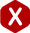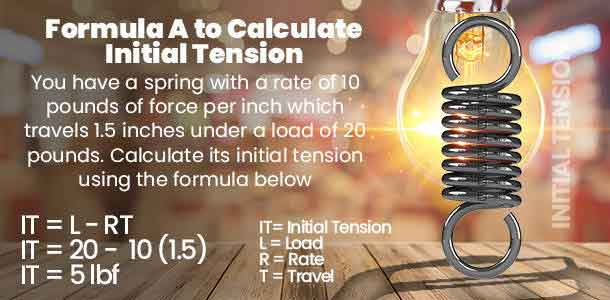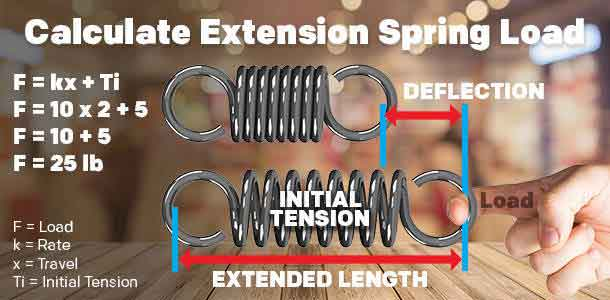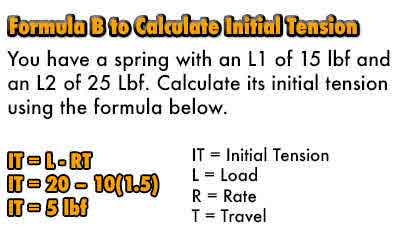<# Initial Tension

#### Definition:The tension already gathered in between an extension spring’s coils to keep the coils together is called initial tension. Initial tension is released once the extension spring is extended to a point where you can see light in between the coils.

Extension spring rate is the constant force your spring exerts per inch of distance traveled. Initial tension will be added to this load in a non-concurring manner. This means that, unlike rate, initial tension will not increment, it will simply be added to the force of your spring rate once. Take a look at the examples shown below for a clearer explanation and the different ways you can calculate initial tension.

#### Initial Tension Formulas & Examples

##### Example A.)

You have an extension spring with a rate (R) of ten pounds per inch and a working load (L) of 20 pounds at a distance traveled (T) of one and a half inches. If you multiply the rate by the distance traveled and subtract the remaining value from the load, you will get the pounds of force of your extension spring’s initial tension as shown in the formula below.

IT = L – R(T)

IT = 20 – 10 * 1.5
IT = 20 – 15
IT = 5 lbf
##### Key Terms
• IT = Initial Tension
• R = Rate
• T = Travel##### Example B.)

You have an extension spring but aren’t sure of the rate. In this case you would use a load 1 and load 2. Measure the amount of force it takes for your spring to travel a certain distance (L1) and do the same at a larger traveled distance (L2). For this example, your L1 will be of fifteen pounds and L2 will be twenty-five pounds. Multiply your load 1 by 2 and subtract load 2 from the remaining product. This will give you the pounds of force of your extension spring’s initial tension as the formula dictates below.

IT = 2(L1) – L2

IT = 2(15) – 25
IT = 30 – 25
IT = 5 lbf
##### Key Terms
• IT = Initial Tension